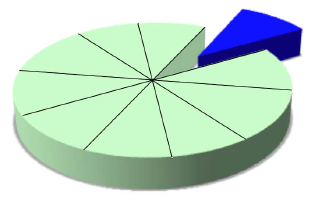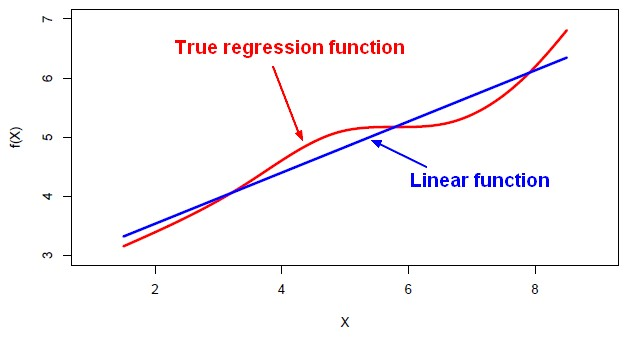# Statistics Learning - Prediction Error (Training versus Test)

The Prediction Error tries to represent the noise through the concept of training error versus test error.

We fit our model to the training set. We take our model, and then we apply it to new data that the model hasn't seen.

In general, because the more data, the bigger the sample size, the more information you have, the lower the error is.

## Metrics

Error calculation:

### Regression

Most of the regression metrics are based on the residual, see Regression Accuracy metrics for a list.

### Classification

Most of the classification metrics are based on the error rate, see Classification Accuracy metrics for a list.

## Type

### Training

Training error is the error we get applying the model to the same data from which we trained.

### Test

Test error is the error that we incur on new data. The test error is actually how well we'll do on future data the model hasn't seen.

## Training vs Test

Training error almost always UNDERestimates test error, sometimes dramatically.

Training error usually UNDERestimates test error when the model is very complex (compared to the training set size), and is a pretty good estimate when the model is not very complex. However, it's always possible we just get too few hard-to-predict points in the test set, or too many in the training set. Then the test error can be LESS than training error, when by chance the test set has easier cases than the training set.

Discover More(Statistics|Data Mining) - (K-Fold) Cross-validation (rotation estimation)

Cross-validation, sometimes called rotation estimation is a resampling validation technique for assessing how the results of a statistical analysis will generalize to an independent new data set. This...Data Mining - (Test|Expected|Generalization) Error

Test error is the prediction error that we incur on new data. The test error is actually how well we'll do on future data the model hasn't seen. The test error is the average error that results from using...Data Mining - Noise (Unwanted variation)

Information from all past experience can be divided into two groups: information that is relevant for the future (“signal”) information that is irrelevant (“noise”). In many cases the factors...Data Mining - Root mean squared (Error|Deviation) (RMSE|RMSD)

Root mean squared (Error|Deviation) in case of regression. The RMSD represents the sample standard deviation of the differences between predicted values and observed values. The RMSE serves to aggregate...Data Mining - Test Set

The test set is a set that is used to validate the model. Test set represent the foresight (unknown data, real data) whereas training Set represents the hindsight. Generally, the test data is created...Data Mining - Training Error

Training error is the prediction error we get applying the model to the same data from which we trained. Training error is much easier to compute than test error. Train error is often lower than test...Machine Learning - (Overfitting|Overtraining|Robust|Generalization) (Underfitting)

A learning algorithm is said to overfit if it is: more accurate in fitting known data (ie training data) (hindsight) but less accurate in predicting new data (ie test data) (foresight) Ie the model...Machine Learning - Linear (Regression|Model)

Linear regression is a regression method (ie mathematical technique for predicting numeric outcome) based on the resolution of linear equation. This is a classical statistical method dating back more...Model Building - ReSampling Validation

Resampling method are a class of methods that estimate the test error by holding out a subset of the training set from the fitting process, and then applying the statistical learning method to those held...R - Feature Selection - Model selection with Direct validation (Validation Set or Cross validation)

Feature selection through model generation and selection through a direct approach with : validation set and cross validation in R We are picking a subset of the observations and put them...